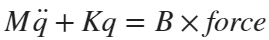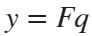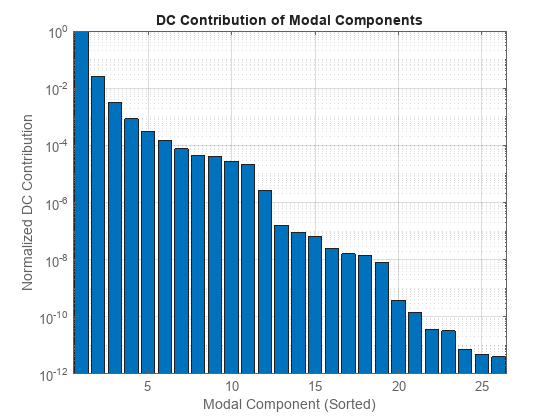# Sparse Modal Truncation of Linearized Structural Beam Model

Since R2023b

This example shows how to eliminate degrees of freedom (DoFs) that are not in the range of interest by using modal truncation model order reduction. In this example, you:

1. Construct a structural model of the beam.

2. Linearize the structural model to obtain a sparse linear model of the beam.

3. Use modal truncation to obtain a reduced-order model.

4. Compare the transient response of the structural model with the linear response of the sparse and reduced models.

This example requires Partial Differential Equation Toolbox™ software.

### Structural Model of Beam

Construct the beam and specify the Young's modulus, Poisson's ratio, and mass density of steel. Specify the tip of the beam using the `addVertex` function.

```gm = multicuboid(0.1,0.005,0.005); E = 210E9; nu = 0.3; rho = 7800; TipVertex = addVertex(gm,"Coordinates",[0.05,0,0.005]);```

Use `createpde` (Partial Differential Equation Toolbox) to construct the structural model and generate the mesh using the `generateMesh` (Partial Differential Equation Toolbox) command.

```sModel = createpde("structural","transient-solid"); sModel.Geometry = gm; msh = generateMesh(sModel);```

Visualize the beam geometry using `pdegplot` (Partial Differential Equation Toolbox).

```figure pdegplot(sModel,"FaceLabels","on"); title("Beam model")```Assign structural properties for the steel beam with the `structuralProperties` (Partial Differential Equation Toolbox) command and fix one end using `structuralBC` (Partial Differential Equation Toolbox).

```structuralProperties(sModel,"YoungsModulus",E,"PoissonsRatio",nu,"MassDensity",rho); structuralBC(sModel,"Face",5,"Constraint","fixed");```

You can calculate the vibration modes of the beam by solving the modal analysis model in a specified frequency range using `solve` (Partial Differential Equation Toolbox). For this beam, the first vibration mode is at 2639 rad/s as confirmed by the Bode plot in the next section of this example.

```firstNF = 2639; Tfundamental = 2*pi/firstNF;```

To model an impulse (knock) on the tip of the beam, apply force for 2% of the fundamental period of oscillation (impulse) using `structuralBoundaryLoad` (Partial Differential Equation Toolbox). Specify the label `force` to use this force as a linearization input.

```Te = 0.02*Tfundamental; structuralBoundaryLoad(sModel,"Vertex",TipVertex,... "Force",[0;0;-100],"EndTime",Te,"Label","force");```

Set initial conditions for the beam model using `structuralIC` (Partial Differential Equation Toolbox).

`structuralIC(sModel,"Velocity",[0;0;0]);`

Compute the impulse response by solving the structural beam model.

```ncycles = 10; tsim = linspace(0,ncycles*Tfundamental,30*ncycles); R1 = solve(sModel,tsim);```

### Structural Model Linearization

For this beam model, you want to obtain a linear model (transfer function) from the force applied at the tip to the z-displacement of the tip.

To do so, first specify the inputs and outputs of the linearized model in terms of the structural model. Here, the input is the force specified with `structuralBoundaryLoad` (Partial Differential Equation Toolbox) and the output is the z degree of freedom of the tip vertex.

```linearizeInput(sModel,"force"); linearizeOutput(sModel,"Vertex",TipVertex,"Component","z");```

Then, use the `linearize` (Partial Differential Equation Toolbox) command to extract the `mechss` model.

`sys = linearize(sModel)`
```Sparse continuous-time second-order model with 1 outputs, 3 inputs, and 3303 degrees of freedom. Model Properties Use "spy" and "showStateInfo" to inspect model structure. Type "help mechssOptions" for available solver options for this model. ```

The linearized model has three inputs corresponding to the x, y, and z components of the force applied to the tip vertex.

`sys.InputName`
```ans = 3×1 cell {'force_x'} {'force_y'} {'force_z'} ```

In the linearized model, select the z component of the force.

`sys = sys(:,3)`
```Sparse continuous-time second-order model with 1 outputs, 1 inputs, and 3303 degrees of freedom. Model Properties Use "spy" and "showStateInfo" to inspect model structure. Type "help mechssOptions" for available solver options for this model. ```

The resultant model is defined by the following set of equations:Visualize the frequency response of the linearized model `sys`.

```w = logspace(0,6,1000); bode(sys,w)```### Model Order Reduction

To perform sparse modal truncation, first create a model order reduction task for the linearized model using `reducespec` with `"modal"` method.

`R = reducespec(sys,"modal");`

For this task, set the frequency range of focus to compute modes up to `5e5` rad/s. Doing so prevents the algorithm from computing all the modes of the sparse model, which can take a long time in some cases.

`R.Options.Focus = [0,5e5];`

Run the model order reduction algorithm using the `process` function. This command computes the derived information you require to generate reduced-order models (ROM), such as modes (poles) and modal components. Additionally, this also helps you avoid recomputing the data in `R` when you call `view` and `getrom`.

`R = process(R);`

Visualize the normalized DC contributions of the modal components.

`view(R,"contrib")`In the frequency range of focus, the model contains 26 modes. Looking at the bar chart, you can can further discard the modes with low DC contribution. For this example, discard the modes with contribution smaller than `1e-6`. This will result in a reduced model with 12 modes.

`rsys = getrom(R,MinDC=1e-6);`

### Compare Transient Response

Compare the frequency response of the linearized model and the reduced-order model.

```figure bodeplot(sys,rsys,w) legend("Linearized full model","Reduced-order model")```The reduced-order model provides a good approximation for the original sparse model in the specified range of interest.

Use `lsim` to simulate the impulse response and compare with the results from the partial differential equation (PDE) model. To limit the error due to linear interpolation of the force between samples, use a step size of `Te/50`. Recall that the force is applied at the beam tip for the short time interval [0 `Te`].

```h = Te/50; t = 0:h:ncycles*Tfundamental; u = zeros(size(t)); u(t<=Te) = -100; y = lsim(sys,u,t); y2 = lsim(rsys,u,t); plot(tsim,R1.Displacement.uz(TipVertex,:)) hold on plot(t,y,t,y2,"--") legend("Structural","Linearized","Reduced",Location="northwest")```The linear response of both sparse and reduced model from `lsim` closely matches the transient simulation of the structural model obtained in the first step.